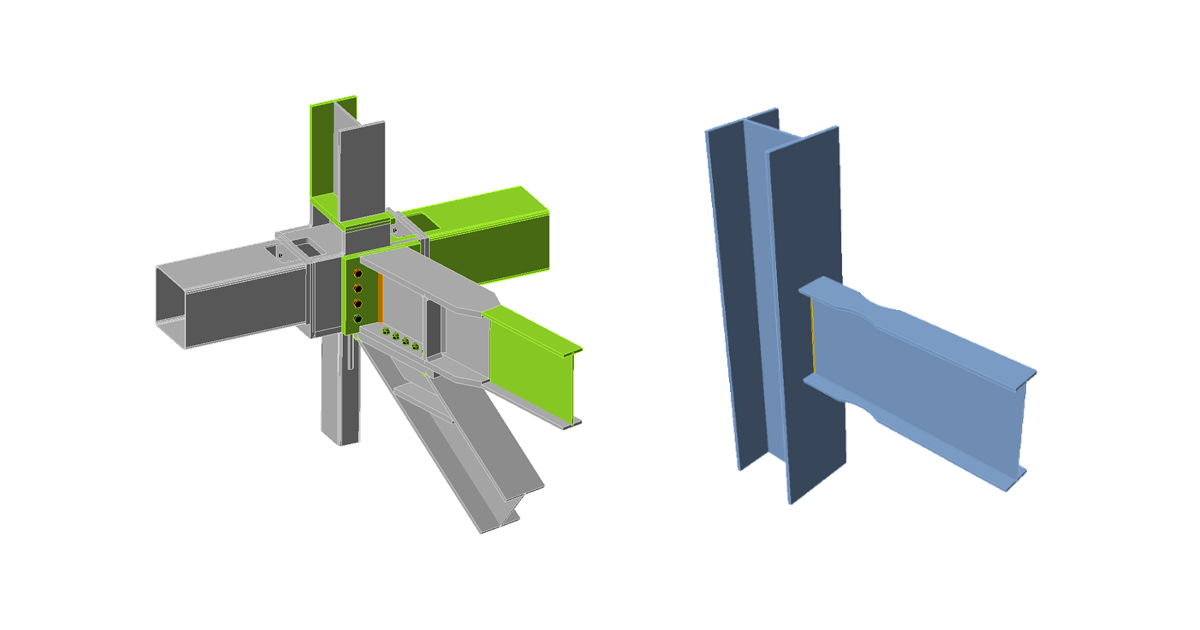### Choose language# Joint classification (EN)

$$Joints are classified according to joint stiffness to: Rigid – joints with insignificant change of original angles between members, Semirigid – joints which are assumed to have the capacity to furnish a dependable and known degree of flexural restraint, Pinned – joints which do not develop bending moments. Joints are classified according to the EN 1993-1-8 – Cl. 5.2.2. Rigid – $$\frac{S_{j,ini} L_b}{E I_b} \ge k_b$$ Semirigid – $$0.5 < \frac{S_{j,ini} L_b}{E I_b} < k_b$$ Pinned – $$\frac{S_{j,ini} L_b}{E I_b} \le 0.5$$ where: Sj,ini – initial stiffness of the joint; the joint stiffness is assumed linear up to the 2/3 of Mj,Rd Lb – theoretical length of the analyzed member; set in member properties E – Young's modulus of elasticity Ib – moment of inertia of the analyzed member kb = 8 for frames where the bracing system reduces the horizontal displacement by at least 80 %; kb = 25 for other frames, provided that in every storey Kb/Kc ≥ 0.1. The value of kb = 25 is used unless the user sets "braced system" in Code setup. Mj,Rd – joint design moment resistance Kb = Ib / Lb Kc = Ic / Lc$$